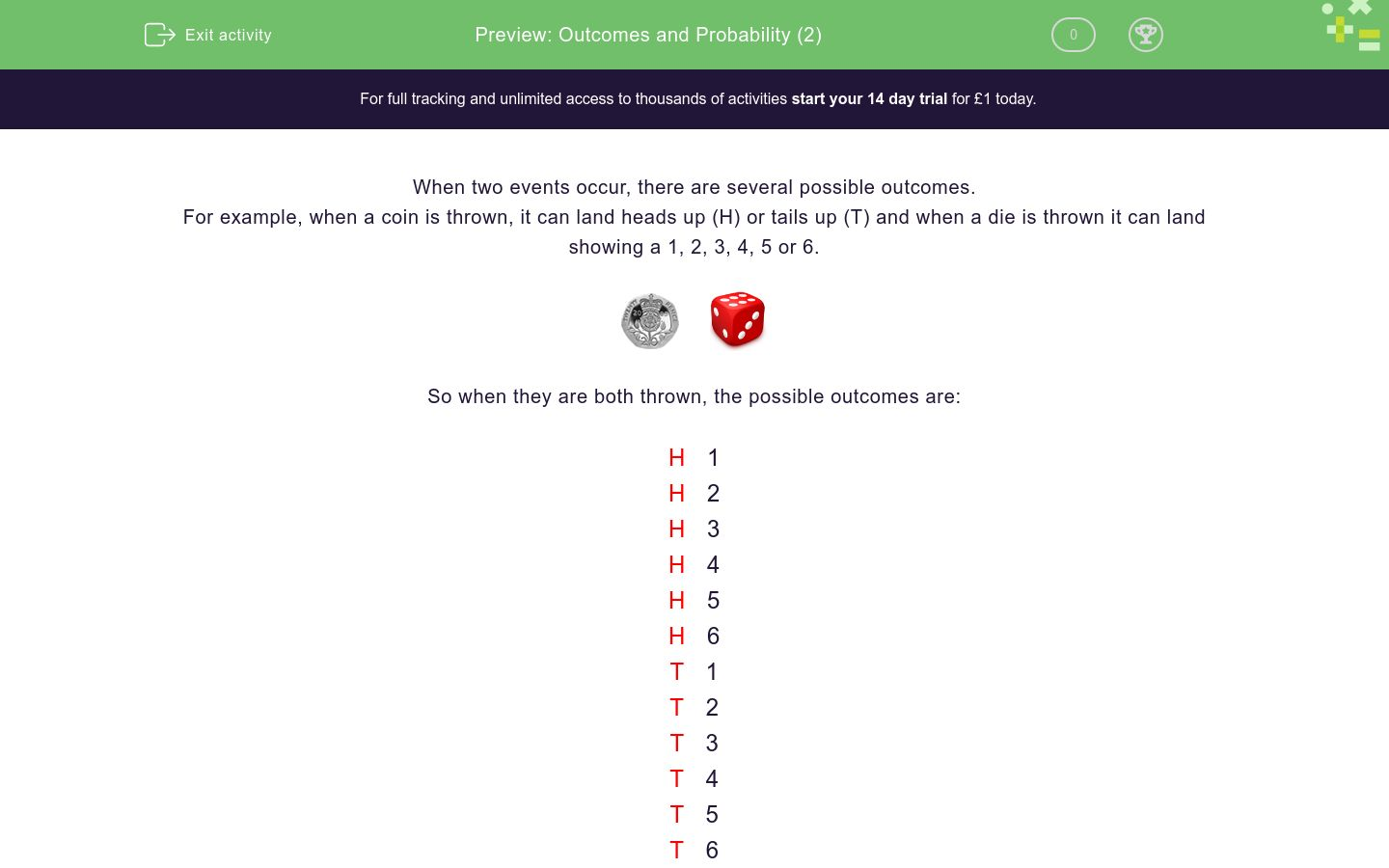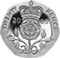# Outcomes and Probability (2)

In this worksheet, students determine the possible outcomes of two events.Key stage:  KS 3

Curriculum topic:   Probability

Curriculum subtopic:   Calculate Theoretical Probability

Difficulty level:### QUESTION 1 of 10

When two events occur, there are several possible outcomes.

For example, when a coin is thrown, it can land heads up (H) or tails up (T) and when a die is thrown it can land showing a 1, 2, 3, 4, 5 or 6.So when they are both thrown, the possible outcomes are:

H   1

H   2

H   3

H   4

5

H   6

1

T   2

3

T   4

5

T   6

There are 12 possible outcomes.

So the probability of getting a head and a 6, P(H6) = 1/12

Make carefully organised, systematic lists when determining all the outcomes.

Do not just write out the outcomes randomly.

One fair coin and one fair die are thrown.Make a list of the possible outcomes on a piece of paper.

In how many outcomes is there an even number on the die?

One fair coin and one fair die are thrown.Make a list of the possible outcomes on a piece of paper.

What is the probability of getting an even number on the die?

(Write your answer with the / symbol, e.g. 2/3 and remember to reduce your fraction to its lowest terms)

One fair coin and one fair die are thrown.Make a list of the possible outcomes on a piece of paper.

In how many outcomes is there a tail and a prime number?

One fair coin and one fair die are thrown.Make a list of the possible outcomes on a piece of paper.

What is the probability of getting a tail on the coin and a prime number on the die?

(Write your answer with the / symbol, e.g. 2/3 and remember to reduce your fraction to its lowest terms)

One fair coin and one fair die are thrown.Make a list of the possible outcomes on a piece of paper.

What is the probability of getting a head on the coin and a factor of 12 on the die?

(Write your answer with the / symbol, e.g. 2/3 and remember to reduce your fraction to its lowest terms)

Two fair dice are thrown.Make a list of the possible outcomes on a piece of paper.

1   1

1   2

1   3

1   4

1   5

1   6

2   1

2   2

2   3

2   4 ...etc

How many outcomes are there?

Two fair dice are thrown.Make a list of the possible outcomes on a piece of paper.

1   1

1   2

1   3

1   4

1   5

1   6

2   1

2   2

2   3

2   4 ...etc

What is the probability of getting two sixes?

(Write your answer with the / symbol, e.g. 2/3 and remember to reduce your fraction to its lowest terms)

Two fair dice are thrown.Make a list of the possible outcomes on a piece of paper.

1   1

1   2

1   3

1   4

1   5

1   6

2   1

2   2

2   3

2   4 ...etc

What is the probability of getting two ones?

(Write your answer with the / symbol, e.g. 2/3 and remember to reduce your fraction to its lowest terms)

Two fair dice are thrown.Make a list of the possible outcomes on a piece of paper.

1   1

1   2

1   3

1   4

1   5

1   6

2   1

2   2

2   3

2   4 ...etc

What is the probability of getting a double (i.e. two ones or two twos or two threes etc.)?

(Write your answer with the / symbol, e.g. 2/3 and remember to reduce your fraction to its lowest terms)

Two fair dice are thrown.Make a list of the possible outcomes on a piece of paper.

1   1

1   2

1   3

1   4

1   5

1   6

2   1

2   2

2   3

2   4 ...etc

What is the probability of getting two even numbers?

(Write your answer with the / symbol, e.g. 2/3 and remember to reduce your fraction to its lowest terms)

• Question 1

One fair coin and one fair die are thrown.Make a list of the possible outcomes on a piece of paper.

In how many outcomes is there an even number on the die?

6
• Question 2

One fair coin and one fair die are thrown.Make a list of the possible outcomes on a piece of paper.

What is the probability of getting an even number on the die?

(Write your answer with the / symbol, e.g. 2/3 and remember to reduce your fraction to its lowest terms)

1/2
• Question 3

One fair coin and one fair die are thrown.Make a list of the possible outcomes on a piece of paper.

In how many outcomes is there a tail and a prime number?

3
• Question 4

One fair coin and one fair die are thrown.Make a list of the possible outcomes on a piece of paper.

What is the probability of getting a tail on the coin and a prime number on the die?

(Write your answer with the / symbol, e.g. 2/3 and remember to reduce your fraction to its lowest terms)

1/4
• Question 5

One fair coin and one fair die are thrown.Make a list of the possible outcomes on a piece of paper.

What is the probability of getting a head on the coin and a factor of 12 on the die?

(Write your answer with the / symbol, e.g. 2/3 and remember to reduce your fraction to its lowest terms)

5/12
• Question 6

Two fair dice are thrown.Make a list of the possible outcomes on a piece of paper.

1   1

1   2

1   3

1   4

1   5

1   6

2   1

2   2

2   3

2   4 ...etc

How many outcomes are there?

36
• Question 7

Two fair dice are thrown.Make a list of the possible outcomes on a piece of paper.

1   1

1   2

1   3

1   4

1   5

1   6

2   1

2   2

2   3

2   4 ...etc

What is the probability of getting two sixes?

(Write your answer with the / symbol, e.g. 2/3 and remember to reduce your fraction to its lowest terms)

1/36
• Question 8

Two fair dice are thrown.Make a list of the possible outcomes on a piece of paper.

1   1

1   2

1   3

1   4

1   5

1   6

2   1

2   2

2   3

2   4 ...etc

What is the probability of getting two ones?

(Write your answer with the / symbol, e.g. 2/3 and remember to reduce your fraction to its lowest terms)

1/36
• Question 9

Two fair dice are thrown.Make a list of the possible outcomes on a piece of paper.

1   1

1   2

1   3

1   4

1   5

1   6

2   1

2   2

2   3

2   4 ...etc

What is the probability of getting a double (i.e. two ones or two twos or two threes etc.)?

(Write your answer with the / symbol, e.g. 2/3 and remember to reduce your fraction to its lowest terms)

1/6
• Question 10

Two fair dice are thrown.Make a list of the possible outcomes on a piece of paper.

1   1

1   2

1   3

1   4

1   5

1   6

2   1

2   2

2   3

2   4 ...etc

What is the probability of getting two even numbers?

(Write your answer with the / symbol, e.g. 2/3 and remember to reduce your fraction to its lowest terms)

1/4
---- OR ----

Sign up for a £1 trial so you can track and measure your child's progress on this activity.

### What is EdPlace?

We're your National Curriculum aligned online education content provider helping each child succeed in English, maths and science from year 1 to GCSE. With an EdPlace account you’ll be able to track and measure progress, helping each child achieve their best. We build confidence and attainment by personalising each child’s learning at a level that suits them.

Get started